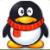# 利用PHP的curl采集数据内存溢出问题咨询

``````public function test(){
ignore_user_abort(true);
set_time_limit(0);

while (true){
\$ch = curl_init();
curl_setopt(\$ch, CURLOPT_HTTPAUTH, CURLAUTH_BASIC);
curl_setopt(\$ch, CURLOPT_RETURNTRANSFER,1);
curl_setopt(\$ch, CURLOPT_SSL_VERIFYPEER, FALSE);
curl_setopt(\$ch, CURLOPT_CUSTOMREQUEST, 'GET');
curl_setopt(\$ch, CURLOPT_URL, 'http://api.****.com/Data');
\$result = null;
\$result = curl_exec(\$ch);
if(\$result === false) {
return curl_error(\$ch);
}
curl_close(\$ch);

\$result = json_decode(\$result, true);
foreach(\$result['data'] as \$key=>\$v){
\$toTime = time();
\$this->db->Query("INSERT INTO `test_data` (`data_oid`, `data_key`, `data_add_time`) VALUES ('\$v[orderID]', '\$v[orderKey]', '\$toTime')");
}
unset(\$result);
sleep(2);
}
}``````

PHP Fatal error:  Allowed memory size of 536870912 bytes exhausted (tried to allocate 56847 bytes) in /var/www/test.php on line 19

19行中就是：\$result = json_decode(\$result, true);    这里，老是报内存溢出。有时候\$result = curl_exec(\$ch);该处也报内存溢出。加了unset(\$result);，还是一样，其中memory_limit默认是128M的，后面由于内存溢出我就加了ini_set('memory_limit', '512M');，把memory_limit改成了512M，后面执行后就是执行的时间比之前128M，时间长点而已，但还是报内存溢出，然后程序中止运行了。各位大牛，帮帮忙，这一般是什么原因，unset也加了，为什么还是报内存溢出。急，

0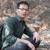``\$this->db->Query("INSERT INTO `test_data` (`data_oid`, `data_key`, `data_add_time`) VALUES ('\$v[orderID]', '\$v[orderKey]', '\$toTime')");``

``````
\$m1 = memory_get_usage();
\$this->db->Query("INSERT INTO `test_data` (`data_oid`, `data_key`, `data_add_time`) VALUES ('\$v[orderID]', '\$v[orderKey]', '\$toTime')");
\$m2 = memory_get_usage();
echo "\n写入数据库之后，内存变化：".(\$m2 - \$m1);
``````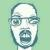0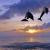\$result内容有多大\$result,是json格式字符串，长度大概有：24599。不算很大啊，都可以执行，但就是执行几个小时后，报内存溢出
0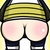00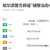00